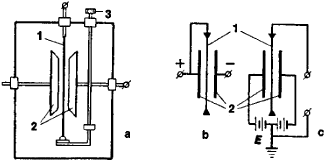# Electrometer

(redirected from Electrometry)
Also found in: Dictionary, Thesaurus, Medical.

## Electrometer

A highly sensitive instrument which measures all or some of the following variables: current, charge, voltage, and resistance. There are two classes of electrometers, mechanical and electronic. The mechanical instruments have been largely replaced by electronic types. See Current measurement, Electrical measurements, Voltage measurement

Mechanical electrometers rely for their operation on the mechanical forces associated with electrostatic fields. Attracted-disk instruments, in which the attractive force between two plates, with a potential difference between them, is measured in terms of the fundamental units of mass and length, are sometimes termed absolute electrometers. They are widely used as electrostatic voltmeters for measuring potentials greater than 1 kilovolt. See Electrical units and standards

The quadrant electrometer consists of a cylindrical metal box divided into quadrants which stand on insulating pillars. Opposite quadrants are connected electrically, and a light, thin metal vane of large area is suspended by a conducting torsion fiber inside the quadrants. An unknown potential is applied across the two quadrant pairs, and electrostatic forces on the vane cause a deflection proportional to the potential. Potentials as low as 10 millivolts can be measured. Small charges and currents can also be measured if the capacitance between vane and quadrants is known. See Electroscope, Electrostatics, Voltmeter

Electronic electrometers utilize some form of electronic amplifier, typically an operational amplifier with a field-effect-transistor input stage to minimize the input current. In the most sensitive applications, problems arise due to drift in the amplifier characteristics and electrical noise present in the circuit components. To obviate these effects, electrometers employing a vibrating capacitor or varactor diodes are used. The signal to be measured is converted to an alternating-current (ac) signal and subsequently amplified by an ac amplifier which is less susceptible to drift and noise. The amplified signal is finally reconverted to direct current (dc). See Transistor

McGraw-Hill Concise Encyclopedia of Physics. © 2002 by The McGraw-Hill Companies, Inc.
The following article is from The Great Soviet Encyclopedia (1979). It might be outdated or ideologically biased.

## Electrometer

an instrument designed to measure differences in electric potentials, small electric charges, very small currents (down to 10–15 ampere), and other electrical quantities in cases where the measuring instrument must consume very little energy. An electrometer is an electrostatic instrument having three electrodes that are generally at different potentials. The most common types are the string and quadrant electrometers, which are used for voltage measurements.

In the simplest string electrometer, the voltage to be measured is fed to a platinum fiber (string) and to fixed electrodes (Figures 1, a and 1, b). The filament is deflected by the force of the electric field, and the displacement of the filament, which is proportional to the magnitude of the quantity being measured, is observed with a microscope. The instrument thus affords fairly high sensitivity. The sensitivity of a string electrometer may be increased by applying an additional voltage to the fixed electrodes (50–100 volts with respect to ground) of the same type (AC or DC) and at the same frequency as the voltage being measured (Figure l,c). The sensitivity of a string electrometer may reach 300–500 mm at 1 volt per m.Figure 1. String electrometer: (a) diagram of physical configuration, (b) and (c) electrical schematics of circuit connection; (1) string (platinum fiber), (2) electrodes, (3) micrometer screw, which adjusts tension on the string (and, thus, the sensitivity of the instrument); (E) source of additional voltage

Quadrant electrometers consist of a movable part in the form of a thin, lightweight, metal vane (the sector plate) with an attached mirror, both of which are suspended on a quartz fiber, together with a stationary part in the form of a cylindrical metal shell divided into four equal sections, or quadrants. When there is a difference between the potentials on the quadrants, electrostatic interaction forces arise between the quadrants and the sector plate that deflect the movable part in one direction or the other. The value of the potential difference on the quadrants can be measured from the angular deflection of the sector plate with a known potential on it; if the potential difference on the quadrants is known, then the potential on the sector plate can be determined. The sensitivity of quadrant electrometers may reach 5,000 mm at 1 volt per m. The binant electrometer, in which the stationary part is divided into two sections, or binants, is a variation of the quadrant electrometer.

### REFERENCES

Kurs elektricheskikh izmerenii, part 1. Edited by V. T. Prytkov and A. V. Talitskii. Moscow-Leningrad, 1960.
Veksler, M. S. Elektrostaticheskie pribory. Moscow-Leningrad, 1964.
Osnovy elektroizmeritel’noi tekhniki. Edited by M. I. Levin. Moscow, 1972.